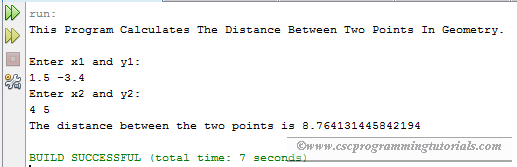# Geometry: Distance Of Two Points Calculator

The program below is the solution to Liang's Introduction to Java Programming (9th Edition) Chapter 2 Exercise 2.19.

Question: Write a program that prompts the user to enter two points (x1, y1) and (x2, y2) and displays their distance between them.

```/**
*
* @Author: Aghatise Osazuwa
* Website: www.cscprogrammingtutorials.com
*
* Exercise 2.19 - Geometry: distance of two points
*
*/

import java.util.Scanner;

public class Ex02_19 {

public static void main(String[] args) {

// Display Program Information
System.out.println("This Program Calculates The Distance Between Two "
+ "Points In Geometry.\n");

// create Scanner
Scanner input = new Scanner(System.in);

// prompt user to enter details
System.out.println("Enter x1 and y1: ");
double x1 = input.nextDouble();
double y1 = input.nextDouble();
System.out.println("Enter x2 and y2: ");
double x2 = input.nextDouble();
double y2 = input.nextDouble();

// calculate distance
double distance = Math.pow(((x2 - x1) * (x2 - x1)) + ((y2 - y1) * (y2 - y1)), 0.5);

//display the result
System.out.println("The distance between the two points is " + distance + "\n");
}
}
```Program output

Click here to see other solutions to Introduction to Java Programming.